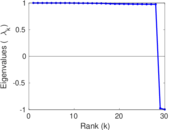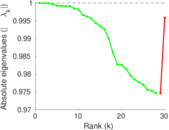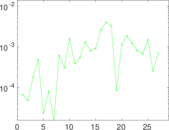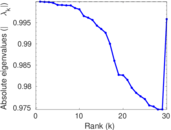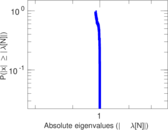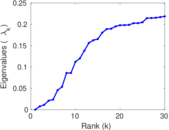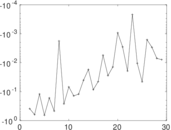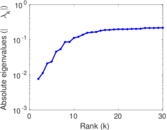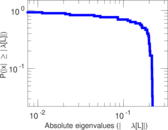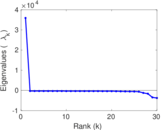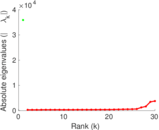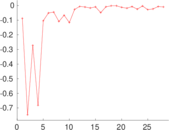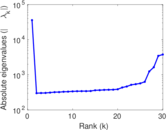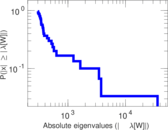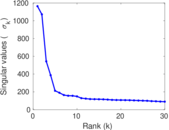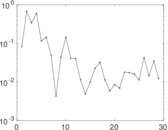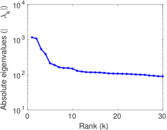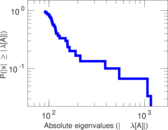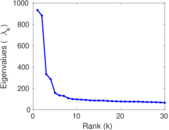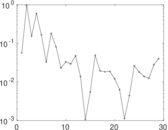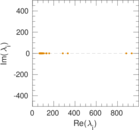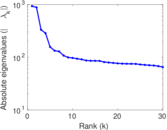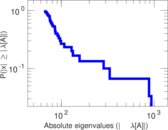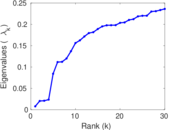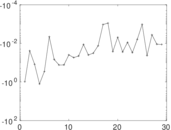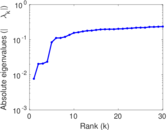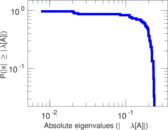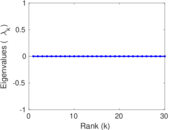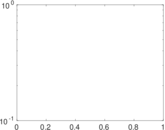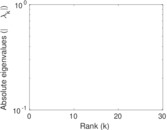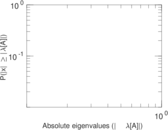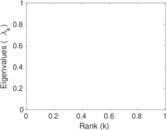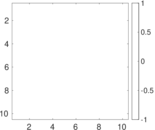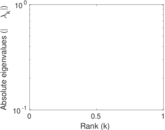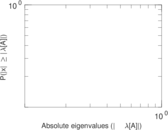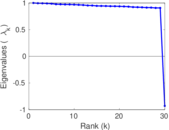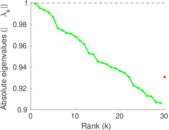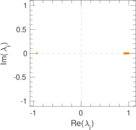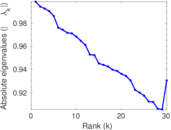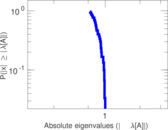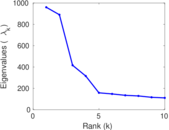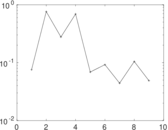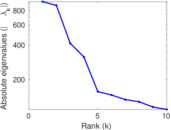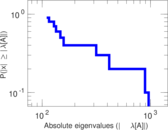This network consists of the wikilinks of the Wikipedia in the Nepali language (ne). Nodes are Wikipedia articles, and directed edges are wikilinks, i.e., hyperlinks within one wiki. In the wiki source, these are indicated with [[double brackets]]. Only pages in the article namespace are included.

 Code `Wne` Internal name `wikipedia_link_ne` Name Wikipedia links (ne) Data source http://dumps.wikimedia.org/ AvailabilityDataset is available for download Consistency checkDataset passed all tests Category Hyperlink network Node meaning Article Edge meaning Wikilink Network format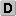Unipartite, directed Edge typeUnweighted, no multiple edges ReciprocalContains reciprocal edges Directed cyclesContains directed cycles Loops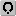Contains loops

## Statistics

 Size n = 36,211 Volume m = 2,941,201 Loop count l = 56 Wedge count s = 1,275,981,932 Cross count x = 1,493,564,851,391,395 Triangle count t = 362,882,218 Square count q = 246,022,008,696 4-Tour count T4 = 1,973,283,583,734 Maximum degree dmax = 8,714 Maximum outdegree d+max = 3,565 Maximum indegree d−max = 8,364 Average degree d = 162.448 Fill p = 0.002 243 07 Size of LCC N = 35,729 Size of LSCC Ns = 22,266 Relative size of LSCC Nrs = 0.614 896 Diameter δ = 11 50-Percentile effective diameter δ0.5 = 2.906 52 90-Percentile effective diameter δ0.9 = 3.976 25 Median distance δM = 3 Mean distance δm = 3.450 33 Gini coefficient G = 0.841 394 Balanced inequality ratio P = 0.147 945 Outdegree balanced inequality ratio P+ = 0.158 929 Indegree balanced inequality ratio P− = 0.152 771 Degree assortativity ρ = +0.155 344 Degree assortativity p-value pρ = 0.000 00 In/outdegree correlation ρ± = +0.772 640 Clustering coefficient c = 0.853 183 Directed clustering coefficient c± = 0.980 537 Operator 2-norm ν = 981.814 Cyclic eigenvalue π = 933.603 Algebraic connectivity a = 0.007 610 67 Spectral separation |λ1[A] / λ2[A]| = 1.078 54 Reciprocity y = 0.780 602 Non-bipartivity bA = 0.917 945 Normalized non-bipartivity bN = 0.004 153 65 Algebraic non-bipartivity χ = 0.008 256 94 Spectral bipartite frustration bK = 2.061 71 × 10−5

## Plots

### Fruchterman–Reingold graph drawing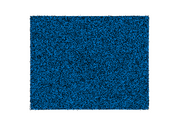### Degree distribution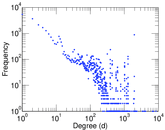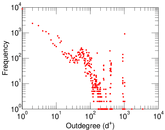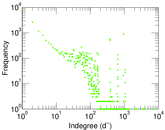### Cumulative degree distribution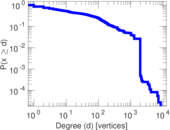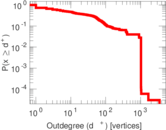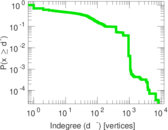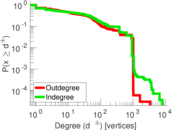### Lorenz curve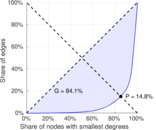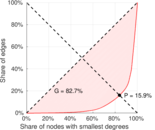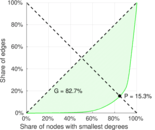### Degree assortativity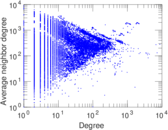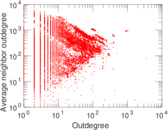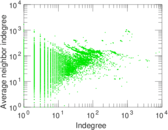### Zipf plot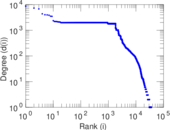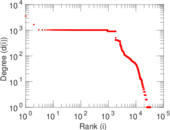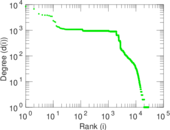### Hop distribution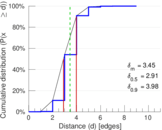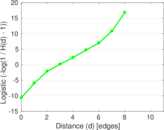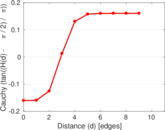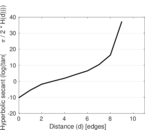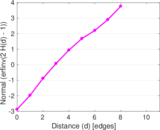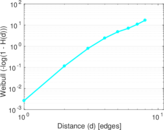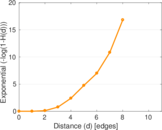### In/outdegree scatter plot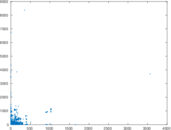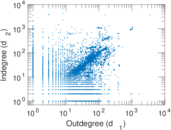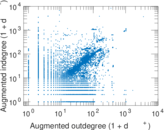### SynGraphy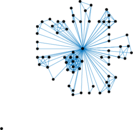### Matrix decompositions plots